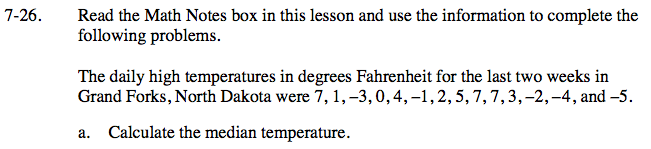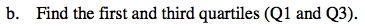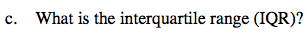Home > CC2 > Chapter 7 > Lesson 7.1.2 > Problem7-26

7-26.
1. 7‑26. Read the Math Notes box in this lesson and use the information to complete the following problems. Homework Help ✎

2. The daily high temperatures in degrees Fahrenheit for the last two weeks in Grand Forks, North Dakota were 7, 1, –3, 0, 4, –1, 2, 5, 7, 7, 3, –2, –4, and –5.

1. Calculate the median temperature.

2. Find the first and third quartiles (Q1 and Q3).

3. What is the interquartile range (IQR)?Refer to the Math Notes box in this lesson.

Order the data from least to greatest, then find the middle of the data set.Now find the median of the lower and upper halves of the data set.The IQR is calculated by subtracting the first quartile from the third quartile

IQR = 7